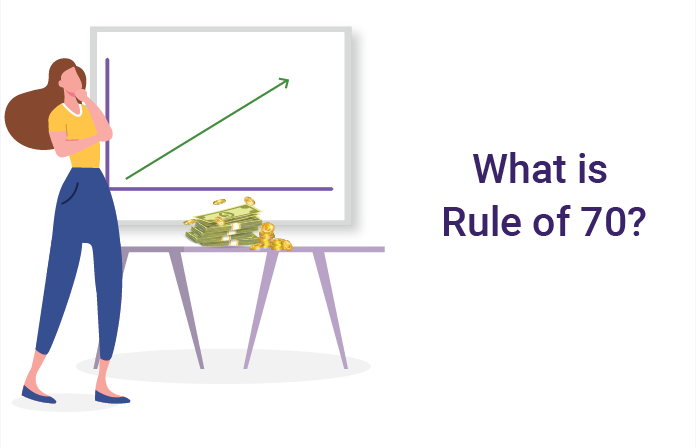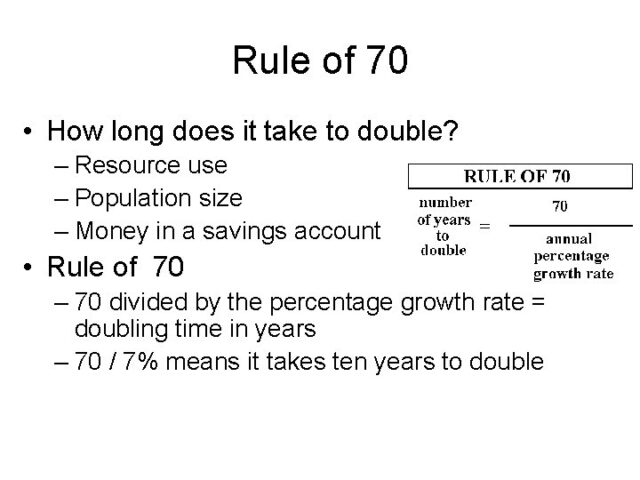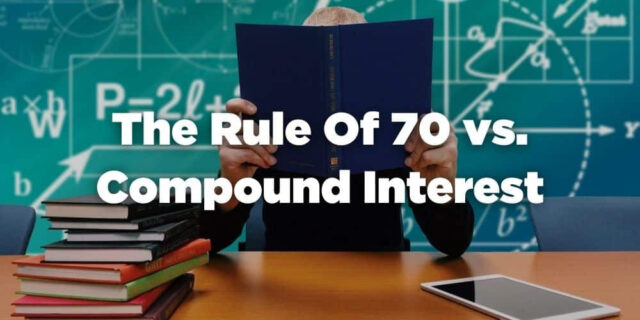# Rule Of 70: What is and How to Implement? [Explained]

For Beginners in the game of investing and trading, the rule of 70 is a big question. To utilize and gain benefits from the rule, you need to answer the most important question, what is the rule of 70. In simple language it can be defined as a formula and concept which tells you how much time it will take your investment to become double. It allows you to calculate months, years, or decades in which your investment will yield a satisfactory amount.

This rule of 70 is calculated on the basis of the current rate of interest your investment is held on. Majorly, the rule is used to compare discrete investing options based on growth rates. It helps the investors to look and analyze the growth period of certain investment options. It gives a prior image of how much time it will take for the investment to give you a double amount in return.## Steps Of Calculating The Rule Of 70 For Your Investment

To calculate the rule of 70 for your own investment, you can use the basic formula and get accurate results. The basic rule can be calculated simply by dividing 70 by the current rate of interest of your investment. You will obtain years in which your investment will get double as per the current rate of interest.All you need is an actual growth rate or rate of interest on your investment. Make sure you recheck your digits to get better and accurate results.

### What Will You Obtain Out Of The Rule Of 70

For the investors using or thinking of using the rule of 70, it should be clear that they will get a rough estimate. This rough estimate will tell you approximately the years in which your investment will double up in the coming future. You can calculate the near future of your investment. This will help you to attain clarity in which investment you will get returns faster. Various investments such as mutual funds or PFs can be judged and analyzed based on this formula and its outcomes. This rule is applicable to a large variety of investments.

Rule of 70 can also be called a simple method to obtain and calculate growth in investment prices exponentially. This process does not require high-order mathematical calculations and highly skilled accountants. However, analyzing and understanding the concept of this rule can be confusing in the beginning. It simply provides an approx value of years in which your current rate of interest or growth rate will take you to its double the investment. The formula and calculations are simple and hence, preferred.

### Larger Scale Application Of Rule Of 70

Rule of 70 is widely used by investors for their small and big investments in daily life. However, the rule of 70 is not limited to your personal investments only. Rule of 70 has a larger scope than this. It can be used to calculate the growth of a country based on the growth rate.Like other investments, a country’s GDP rate is also its citizen’s investment. Therefore, if you are wondering that in how much time the GDP of your country will grow, use Rule of 70. Calculation of Rule of 70 for GDP growth can provide you with estimated years in which the current capital of your country will get doubled at the current rate of growth. You can simply use the same formula of the rule. And divide 70 with the current growth rate of the country’s GDP this time. It will provide an estimated value of years.

### Comparing Rule Of 70 With Actual Growth Scenario

Before getting completely dependent on the data acquired by using the rule of 70, it is important to understand that rule of 70 is an estimation-based formula. It only provides an estimated value in years for doubling your investment. Also, it is applicable for investments with a regular and constant rate of interest. If the rate of interest varies over a span, the rule of 70 will not be applicable.There are many investments that have a linearly growing rate of interest where the rule of 70 will fail to perform accurate results. The investors looking for the estimated amount of time and don’t want to indulge in heavy mathematical calculations, the rule of 70 is best suitable. Also, investments with a constant and stable rate of interest for a long time can also benefit from the rule of 70. Therefore, before comparing your actual growth in an investment, make sure that the rule of 70 is accurately applied.

### Why Is The Rule Of 70 Different From Compound Interest

For beginners, working of the rule of 70 can look familiar to working of compound interest. However, both the concepts are different from each other. To understand the difference between the rule of 70 and compound interest, it is important to look at both the terms individually.Compound interest is a concept used for longer investment returns. It is calculated on a certain fixed value, also called principal amount. This principle amount is added on with an amount on the basis of interest rate. Compounding is referred to a technique of adding interest to the constantly growing amount in addition to the principal amount.

Investments with frequent compounding give a better rate of interest on the principal amount than less compounding. To study and use different rules of doubling your investment amount, compound interest is important. For the investors looking for earning interests without reinvesting, the time period taken to double the initial value will be more than double that of those who wish to reinvest.

On the other hand, for the rule of 70 to work, you need to keep the principal amount and rate of interest common. Only then will it give accurate and satisfactory results. Therefore, before applying any of the concepts, you need to look for your investment options and choices.

### Limitations Of Rule Of 70

Rule of 70 has huge applications. However, it comes with certain limitations in working major limitation of the rule of 70 is accuracy. Rule of 70 is based on estimations. Giving the investors an idea of the years it will take to double their investment amount. But it does not provide accurate data and the number of years. Also, another limitation of the rule is that you need a constant and correct annual interest rate to calculate the rule of 70. The formula is entirely dependent and applicable on a constant rate of interest.

With investments having varied interest rates over a time span, the rule proves to be inaccurate. Even the investments with different principal amounts, the rule of 70 will not work. It can be used for the investments to be made in a limited amount of time. In a limited amount of principle value with a constant rate of interest, the rule works well. Another limitation of the rule is you can not calculate the number of years it will need to gain a certain amount. It will only provide you the probably years in which your investment will double up.

### Conclusion

Rule of 70 is a simple, effective, and efficient rule to calculate growth years of investments. For the investors looking for an easy way to calculate growth in years in which the principal amount will double itself with the current rate of interest, the rule of 70 is the one. It can be done without professional help or extraordinary knowledge of accounts. Investors can calculate doubling years using the rule of 70 on their own.

With this rule, you can calculate doubling years for large and small-scale investments. It can provide you with probable years in which you can expect your investment to double. This can also help you to change your rate or principal amount to achieve the desired amount sooner. You can increase the rate of interest, which will decrease the doubling years, and you can get double investment within a limited time.

Also Read: Best ways to earn money.

• ##### How to use Rule of 70?

This rule is used to determine the time needed to double any number according to the growth rate. All you have to do is to divide 70 by the growth rate of the number. The result will be the number of years it takes to double.

• ##### Explain Rule of 72 in investing?

This rule is used to determine the time needed to double any number according to the annual rate of return. All you have to do is to divide 72 by the annual rate of return. The result will be the number of years it takes to double.

• ##### How much time will it take to double my money invested at the compound rate of 5% annually?

According to the compound interest formula, the money will be doubled in 20 years if compounded annually at 5%.

• ##### Can you apply Rule of 72 to compound interest?

Fortunately, you can. The rule of 72 can be applied to compound interest. Also, the rule is almost correct for interest rates between 6% and 10%.

• ##### What are the ways to double your money?

To double your money, you may have to invest it, reinvest it, use penny stocks, or buying products on margin. Other than this, you can also use the tax-advantaged accounts or retirement account provided by the job providers.